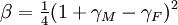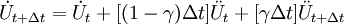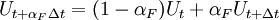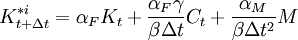Generalized Alpha Method - OpenSeesWiki

# Generalized Alpha Method

Jump to: navigation, search

This command is used to construct a Generalized α integration object. This is an implicit method that like the HHT method allows for high frequency energy dissipation and second order accuracy, i.e. Δt2. Depending on choices of input parameters, the method can be unconditionally stable.

 integrator GeneralizedAlpha $alphaM$alphaF <$gamma$beta>

 $alphaM αM factor$alphaF αF factor $gamma γ factor$beta β factor

EXAMPLE:

integrator GeneralizedAlpha 1.0 0.8

NOTES:

1. αF and αM are defined differently that in the paper, we use αF = (1 − αf) and αM = (1 − γm) where αf and αm are those used in the paper.
2. Like Newmark and all the implicit schemes, the unconditional stability of this method applies to linear problems. There are no results showing stability of this method over the wide range of nonlinear problems that potentially exist. Experience indicates that the time step for implicit schemes in nonlinear situations can be much greater than those for explicit schemes.
3. αM = 1.0,αF = 1.0 produces the Newmark Method.
4. αM = 1.0 corresponds to the HHT method.
5. The method is second-order accurate provided$\gamma = \tfrac{1}{2} + \alpha_M - \alpha_F$
6. The method is unconditionally stable provided$\alpha_M >= \alpha_F >= \tfrac{1}{2}, \beta>=\tfrac{1}{4} +\tfrac{1}{2}(\gamma_M - \gamma_F)$
7. γ and β are optional. The default values ensure the method is unconditionally stable, second order accurate and high frequency dissipation is maximized.

The defaults are:$\gamma = \tfrac{1}{2} + \gamma_M - \gamma_F$

and$\beta = \tfrac{1}{4}(1 + \gamma_M - \gamma_F)^2$

REFERENCES

J. Chung, G.M.Hubert. "A Time Integration Algorithm for Structural Dynamics with Improved Numerical Dissipation: The Generalized-α Method" ASME Journal of Applied Mechanics, 60, 371:375, 1993.

THEORY:

The Generalized α method (sometimes called the α method) is a one step implicit method for solving the transient problem which attempts to increase the amount of numerical damping present without degrading the order of accuracy. In the HHT method, the same Newmark approximations are used:$U_{t+\Delta t} = U_t + \Delta t \dot U_t + [(0.5 - \beta) \Delta t^2] \ddot U_t + [\beta \Delta t^2] \ddot U_{t+\Delta t}$$\dot U_{t+\Delta t} = \dot U_t + [(1-\gamma)\Delta t] \ddot U_t + [\gamma \Delta t ] \ddot U_{t+\Delta t}$

but the time-discrete momentum equation is modified:$R_{t + \alpha_M \Delta t} = F_{t+\Delta t}^{ext} - M \ddot U_{t + \alpha_M \Delta t} - C \dot U_{t+\alpha_F \Delta t} - F^{int}(U_{t + \alpha_F \Delta t})$

where the displacements and velocities at the intermediate point are given by:$U_{t+ \alpha_F \Delta t} = (1 - \alpha_F) U_t + \alpha_F U_{t + \Delta t}$$\dot U_{t+\alpha_F \Delta t} = (1-\alpha_F) \dot U_t + \alpha_F \dot U_{t + \Delta t}$$\ddot U_{t+\alpha_M \Delta t} = (1-\alpha_M) \ddot U_t + \alpha_M \ddot U_{t + \Delta t}$

Following the methods outlined for Newmarks method, linearization of the nonlinear momentum equation results in the following linear equations:$K_{t+\Delta t}^{*i} d U_{t+\Delta t}^{i+1} = R_{t+\Delta t}^i$

where$K_{t+\Delta t}^{*i} = \alpha_F K_t + \frac{\alpha_F \gamma}{\beta \Delta t} C_t + \frac{\alpha_M}{\beta \Delta t^2} M$

and$R_{t+\Delta t}^i = F_{t + \Delta t}^{ext} - F(U_{t + \alpha F \Delta t}^{i-1})^{int} - C \dot U_{t+\alpha F \Delta t}^{i-1} - M \ddot U_{t+ \alpha M \Delta t}^{i-1}$

The linear equations are used to solve for$U_{t+\alpha F \Delta t}, \dot U_{t + \alpha F \Delta t} \ddot U_{t+ \alpha M \Delta t}$. Once convergence has been achieved the displacements, velocities and accelerations at time t + Δt can be computed.

Code Developed by: fmk